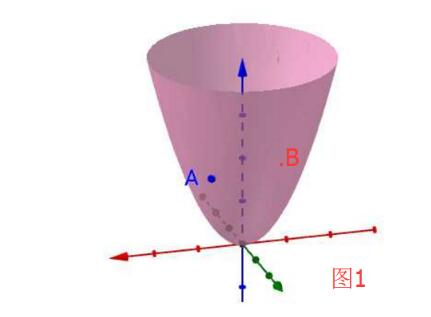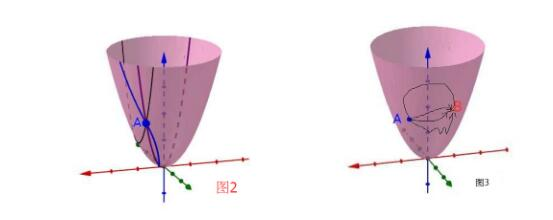# 二元函数偏导数公式_多元函数的偏导数、方向导数、梯度以及微分之间的关系思考...(1)函数在A点的趋势变化。

(2)函数从A到B的变化的量。

(3)函数降维时候的变化，比如固定y，将二元函数看成一个一元函数来让x单独变化，又会产生什么变化。(1)方向导数：本质就是函数在A点无数个切线的斜率的定义。每一个切线都代表一个变化的方向。

(2)梯度：函数在A点无数个变化方向中变化最快的那个方向。

(3)全微分：函数从A点到B点变化的量(其实是取一个无穷小的变化的量)。

(4)偏导：多元函数降维时候的变化，比如二元函数固定y，只让x单独变化，从而看成是关于x的一元函数的变化来研究。

2.1 偏导

${f_x}(x{\rm{y}}) = \mathop {\lim }\limits_{\Delta x \to 0} \frac{{f(x + \Delta x,y) - f(x,y)}}{{\Delta x}}$

2.2 方向导数

$\begin{array}{l} \frac{{\partial f}}{{\partial L}} = {f_x}\cos \alpha + {f_y}\cos \beta \\ \cos \alpha = \frac{{\overrightarrow {A{B_x}} }}{{\left| {\overrightarrow {AB} } \right|}}{\kern 1pt} {\kern 1pt} {\kern 1pt} {\kern 1pt} {\kern 1pt} {\kern 1pt} {\kern 1pt} {\kern 1pt} {\kern 1pt} {\kern 1pt} {\kern 1pt} {\kern 1pt} {\kern 1pt} {\kern 1pt} {\kern 1pt} {\kern 1pt} {\kern 1pt} {\kern 1pt} \cos \beta = \frac{{\overrightarrow {A{B_y}} }}{{\left| {\overrightarrow {AB} } \right|}}\\ \overrightarrow {AB} = ({x_1} - {x_2},{y_1} - {y_2}) \end{array}$

2.3 梯度

$gradA = ({f_x}(A),{f_y}(A),{f_z}(A))$

2.4 全微分

2.5 切平面

11-23498
12-221826
04-273万+
10-301万+
01-171万+
03-312万+
10-06709
12-173549
12-22531
06-108391
01-171万+
12-15743
12-222265
09-161187
10-29724
08-211883
12-202743
01-091万+
09-22785

### “相关推荐”对你有帮助么？

•非常没帮助
•没帮助
•一般
•有帮助
•非常有帮助被折叠的  条评论 为什么被折叠?到【灌水乐园】发言rayyangul

¥2 ¥4 ¥6 ¥10 ¥20余额支付 (余额：-- )扫码支付获取中扫码支付点击重新获取扫码支付1.余额是钱包充值的虚拟货币，按照1:1的比例进行支付金额的抵扣。
2.余额无法直接购买下载，可以购买VIP、C币套餐、付费专栏及课程。余额充值